Updating search results...

# 59 Results

View
Selected filters:
• equations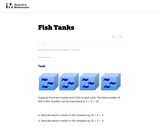Unrestricted Use
CC BY
Rating
0.0 stars

This is a task from the Illustrative Mathematics website that is one part of a complete illustration of the standard to which it is aligned. Each task has at least one solution and some commentary that addresses important asects of the task and its potential use. Here are the first few lines of the commentary for this task: Suppose there are 4 tanks and 3 fish in each tank. The total number of fish in this situation can be expressed as $4 \times 3 = 12$. Describe what is m...

Subject:
Mathematics
Material Type:
Activity/Lab
Provider:
Illustrative Mathematics
Provider Set:
Illustrative Mathematics
Author:
Illustrative Mathematics
08/13/2013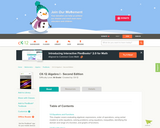Conditional Remix & Share Permitted
CC BY-NC-SA
Rating
0.0 stars

A work in progress, CK-12's Algebra I Second Edition is a clear presentation of algebra for the high school student. Topics include: Equations and Functions, Real Numbers, Equations of Lines, Solving Systems of Equations and Quadratic Equations.

Subject:
Mathematics
Material Type:
Textbook
Provider:
CK-12 Foundation
Provider Set:
CK-12 FlexBook
Author:
Andrew
Annamaria
Anne
Eve
Farbizio
Gloag
Rawley
12/03/2010Rating
0.0 stars

In this lesson, students write and evaluate algebraic expressions at specific values. They will also use variables to represent numbers and write expressions when solving a real-world or mathematical problem.

Subject:
Mathematics
Material Type:
Activity/Lab
Lesson Plan
Provider:
Utah Education Network
Author:
Utah LessonPlans
02/26/2019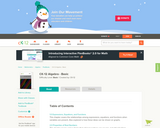Conditional Remix & Share Permitted
CC BY-NC-SA
Rating
0.0 stars

CK-12 Foundation's Basic Algebra FlexBook is an introduction to the algebraic topics of functions, equations, and graphs for middle-school and high-school students.

Subject:
Mathematics
Material Type:
Textbook
Provider:
CK-12 Foundation
Provider Set:
CK-12 FlexBook
Author:
Farbizio, Annamaria
Gloag, Andrew
Gloag, Anne
Kramer, Melissa
09/21/2010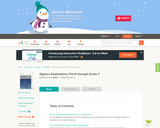Conditional Remix & Share Permitted
CC BY-NC-SA
Rating
0.0 stars

CK-12 Algebra Explorations is a hands-on series of activities that guides students from Pre-K to Grade 7 through algebraic concepts.

Subject:
Mathematics
Material Type:
Activity/Lab
Teaching/Learning Strategy
Provider:
CK-12 Foundation
Provider Set:
CK-12 FlexBook
Author:
Carol Â­ Findell, Mary Â­Cavanagh, Carole Â­Greenes
02/23/2012Rating
0.0 stars

Using tiles to represent variables and constants, learn how to represent and solve algebra problem. Solve equations, substitute in variable expressions, and expand and factor. Flip tiles, remove zero pairs, copy and arrange, and make your way toward a better understanding of algebra.

Subject:
Math 1
Math 2
Math 3
Mathematics
Material Type:
Interactive
Provider:
National Council of Teachers of Mathematics
Author:
Illuminations
02/26/2019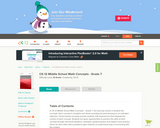Conditional Remix & Share Permitted
CC BY-NC-SA
Rating
0.0 stars

CK-12 Middle School Math Concepts for seventh grade provides a complete textbook. It presents topics including algebraic thinking, patterns, decimals, decimal operations, fractions, fraction operations, integers, integer operations, ratios, rates, proportions, percents, percent applications, equations, solving equations, inequalities, functions, graphing functions, geometry, plane geometry, solid geometry, area, perimeter, surface area, volume, statistics including mean, median, mode and range, graphing and types of graphs, and probability.

Subject:
Mathematics
Material Type:
Textbook
Provider:
CK-12 Foundation
Provider Set:
CK-12 FlexBook
Author:
Jen Kershaw
11/30/2012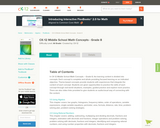Conditional Remix & Share Permitted
CC BY-NC
Rating
0.0 stars

In CK-12 Middle School Math Concepts Grade 8, the learning content is divided into concepts. Each concept is complete and whole providing focused learning on an indicated objective. Theme-based concepts provide students with experiences that integrate the content of each concept. Students are given opportunities to practice the skills of each concept through real-world situations, examples, guided practice and explore more practice. There are also video links provided to give students an audio/visual way of connecting with the content.

Subject:
Mathematics
Material Type:
Full Course
Lesson Plan
Textbook
Unit of Study
Provider:
CK-12 Foundation
Provider Set:
CK-12 FlexBook
12/06/2018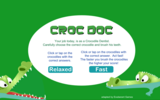Rating
0.0 stars

In this interactive math game, students will learn division facts while brushing the teeth of crocodiles. Students can choose from two different levels, easy and super. Then within each level they can choose from six different fact levels to play. Once they complete the game they can play again.

Subject:
Mathematics
Material Type:
Interactive
Provider:
Sheppard Software
Author:
Sheppard Software
02/26/2019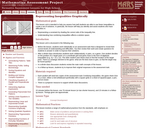Only Sharing Permitted
CC BY-NC-ND
Rating
0.0 stars

This lesson unit is intended to help teachers assess how well students are able to use linear inequalities to create a set of solutions. In particular, the lesson will help teachers identify and assist students who have difficulties in: representing a constraint by shading the correct side of the inequality line; and understanding how combining inequalities affects a solution space.

Subject:
Mathematics
Material Type:
Assessment
Lesson Plan
Provider:
Shell Center for Mathematical Education
Provider Set:
Mathematics Assessment Project (MAP)
07/31/2019Rating
0.0 stars

Students determine how far a brother and sister travel before they catch up with one another. The mathematics task is intended to be a problem or question that encourages the use of mathematical practices. The dialogue is meant to show how students might engage in the mathematical practices as they work on the task.

Subject:
Math 1
Math 2
Math 3
Mathematics
Material Type:
Lesson Plan
Provider:
Education Development Center, Inc.
Author:
Education Development Center, Inc.
02/26/2019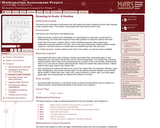Only Sharing Permitted
CC BY-NC-ND
Rating
0.0 stars

This lesson unit is intended to help assess how well students are able to interpret and use scale drawings to plan a garden layout. This involves using proportional reasoning and metric units.

Subject:
Algebra
Geometry
Mathematics
Material Type:
Assessment
Lesson Plan
Provider:
Shell Center for Mathematical Education
Provider Set:
Mathematics Assessment Project (MAP)
06/24/2019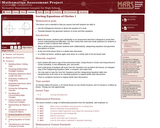Only Sharing Permitted
CC BY-NC-ND
Rating
0.0 stars

This lesson unit is intended to help teachers assess how well students are able to: use the Pythagorean theorem to derive the equation of a circle; and translate between the geometric features of circles and their equations.

Subject:
Geometry
Mathematics
Material Type:
Assessment
Lesson Plan
Provider:
Shell Center for Mathematical Education
Provider Set:
Mathematics Assessment Project (MAP)
07/31/2019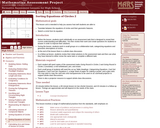Only Sharing Permitted
CC BY-NC-ND
Rating
0.0 stars

This lesson unit is intended to help teachers assess how well students are able to: translate between the equations of circles and their geometric features; and sketch a circle from its equation.

Subject:
Geometry
Mathematics
Material Type:
Assessment
Lesson Plan
Provider:
Shell Center for Mathematical Education
Provider Set:
Mathematics Assessment Project (MAP)
07/31/2019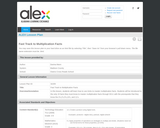Rating
0.0 stars

Students will learn how to use tricks to master multiplication facts. Students will be introduced to only 15 facts they must know to master multiplication facts through 9X11 with the prerequisite that they know the 0's,2's,5's,10's, and 11's.

Subject:
Mathematics
Material Type:
Lesson Plan
Provider:
Alabama Learning Exchange
Author:
Davina Mann
02/26/2019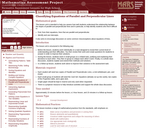Only Sharing Permitted
CC BY-NC-ND
Rating
0.0 stars

This lesson unit is intended to help teachers assess how well students are able to understand the relationship between the slopes of parallel and perpendicular lines and, in particular, to help identify students who find it difficult to: find, from their equations, lines that are parallel and perpendicular; and identify and use intercepts. It also aims to encourage discussion on some common misconceptions about equations of lines.

Subject:
Mathematics
Material Type:
Assessment
Lesson Plan
Provider:
Shell Center for Mathematical Education
Provider Set:
Mathematics Assessment Project (MAP)
07/31/2019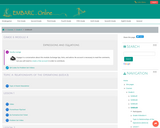Conditional Remix & Share Permitted
CC BY-NC-SA
Rating
0.0 stars

Grade 6 Module 4: Expressions and Equations. Contains 34 Lessons.

Subject:
Mathematics
Material Type:
Module
Provider:
EMBARC.Online
08/13/2019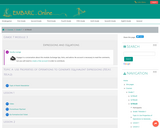Conditional Remix & Share Permitted
CC BY-NC-SA
Rating
0.0 stars

Grade 7 Module 3: Expressions and Equations. Contains 26 Lessons.

Subject:
Mathematics
Material Type:
Module
Provider:
EMBARC.Online
08/13/2019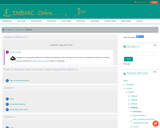Conditional Remix & Share Permitted
CC BY-NC-SA
Rating
0.0 stars

Grade 8 Module 4: Linear Equations. Contains 31 Lessons.

Subject:
Mathematics
Material Type:
Module
Provider:
EMBARC.Online
08/13/2019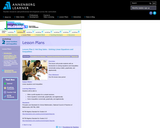Rating
0.0 stars

This lesson will provide students with an introduction to solving equations and inequalities numerically (using a table), graphically, and algebraically.

Subject:
Math 1
Math 3
Mathematics
Material Type:
Activity/Lab
Lesson Plan
Provider:
Annenberg Learner
Author:
Annenberg Learner